# Cauchy problem, numerical methods for ordinary differential equations

A Cauchy problem is a problem of determining a function (or several functions) satisfying a differential equation (or system of differential equations) and assuming given values at some fixed point. Let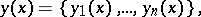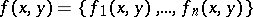be vector-functions defined and continuous, respectively, on a closed intervaland in a closed domain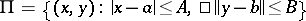, whereis some norm in the finite-dimensional space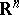. With this notation, one writes the Cauchy problem for a system of first-order ordinary differential equations as follows: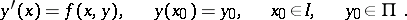(1)

By a suitable choice of new unknown functions one can reduce the Cauchy problem for any system of ordinary differential equations (of arbitrary order) to this form.

Problem (1) has a solution if the functionis continuous in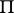. A sufficient condition for the solution to be unique is that the Osgood condition: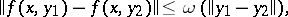(2)

where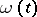is a function such that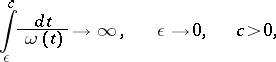or the stronger Lipschitz condition: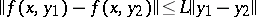(3)

hold. The number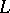is called the Lipschitz constant. Ifis continuously differentiable with respect to, a possible value for the Lipschitz constantis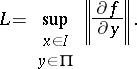(4)

In various cases, where the Lipschitz constant (4) is too large, a successful solution of the Cauchy problem by numerical methods requires special numerical techniques, despite of the fact that, theoretically speaking, the problem is uniquely solvable. This happens, in particular, if the eigen values of the matrix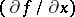are "widely scattered" , i.e. the largest eigen value is hundreds — or even thousands — of times larger than the smallest. Such systems of differential equations are called stiff systems, and the corresponding problems are stiff Cauchy problems. One "source" of stiff systems is the reduction of a partial differential equation to a system of ordinary differential equations, e.g. via the method of lines.

Numerical methods for ordinary differential equations normally consist of one or more formulas defining relations for the function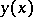to be found at a discrete sequence of points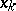,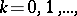the set of which is called a grid. The foundations of numerical methods in general, and of those for differential equations in particular, were laid by y of an elastic system','../s/s087010.htm','Siegel disc','../s/s110120.htm','Theta-function','../t/t092600.htm','Trigonometric series','../t/t094240.htm','Two-term congruence','../t/t094620.htm','Umbral calculus','../u/u095050.htm','Variation of constants','../v/v096160.htm','Variational calculus','../v/v096190.htm','Variational calculus, numerical methods of','../v/v096210.htm','Venn diagram','../v/v096550.htm','Zeta-function','../z/z099260.htm')" style="background-color:yellow;">L. Euler. One of the simplest methods for solving the Cauchy problem is named after him. This method is as follows. Expand the solution of problem (1) in a Taylor series about the point: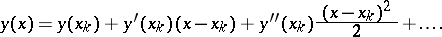If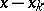is small in absolute value, then, dropping terms of order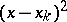and higher, one obtains an approximate equality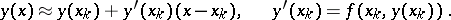The approximate solution may now be evaluated at the pointby the formula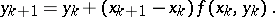This is Euler's method.

Numerical methods were subsequently improved to a considerable degree. This development led in two main directions: methods later known as Runge–Kutta methods (cf. Runge–Kutta method), and linear multi-step methods, the most important of which is the Adams method.

One of the advantages of Runge–Kutta methods is that the algorithms that they produce are uniform, i.e. they remain unchanged upon passage from one grid point to another. In addition, the elementary step length of integration can be modified in Runge–Kutta methods, according to the required computational accuracy, without significantly complicating the algorithm itself (see Kutta–Merson method; Runge rule). Fairly reliable two-sided methods have been developed on the basis of these methods. A major shortcoming is that, in order to compute an approximate solution at one point of the grid, one has to evaluate several values of the (right-hand side) term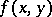of the differential equation (1). This implies — particularly in equations with complicated right-hand side terms — a considerable increase in computation time.

In linear multi-step methods, including the Adams method, one has to compute the right-hand side term at one grid point only. This is the main advantage of these methods. However, in order to begin computing with a linear multi-step formula, one first has to calculate additional "initial values" . Because of this, the algorithm is not self-starting: The first few values must be computed using other formulas. A more serious shortcoming of linear multi-step methods is that the step length of integration cannot be simply altered: One must use grids of constant step length.

Linear multi-step methods have provided the basis for the development of what are known as predictor-corrector methods, comprising a pair of linear multi-step formulas, one of which (the predictor) is usually explicit, while the other (the corrector) is implicit; e.g. the predictor might be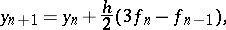and the corrector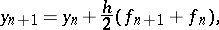wherePredictor-corrector methods are successfully applied in the solution of stiff systems of ordinary differential equations.

Irrespective of the fact that higher-order differential equations are formally reducible to systems of first-order equations, methods adapted to the actual form of the differential equation are sometimes much more effective. In this connection, linear multi-step methods have been developed which utilize higher-order derivatives, such as the Störmer method.